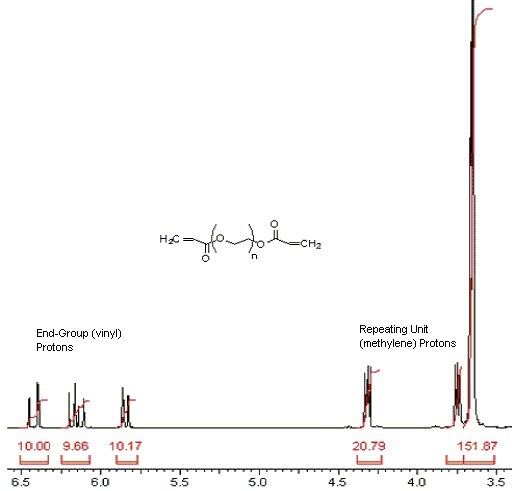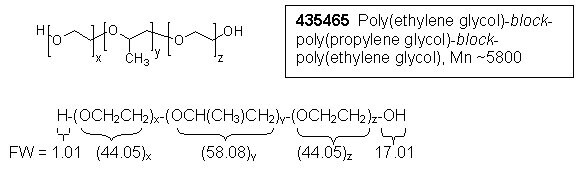Polymer Analysis in Materials Science

Polymer Analysis by NMR

One of the challenges polymer scientists face is molecular weight (avg. chain length) determination of their materials. While membrane osmometry, gel permeation chromatography, viscosity analysis, and mass spectrometry are typically used for molecular weight determination, the techniques can be time consuming, inaccurate for the molecular weight ranges involved, or require specialized instrumentation. End group analysis by NMR offers an easy alternative method using an instrument commonly found in many analytical labs. In addition, NMR analysis can also be used to accurately determine monomer ratios for various copolymer molecules.

Scientists at Sigma-Aldrich routinely determine number-average molecular weight (Mn) by 1H NMR end-group analysis for polymers having Mn values under 3,000. Sensitivity of the instrument and the subsequent ability to detect end-group protons will determine the upper limit that can be measured. The method relies on a few simple needs.

• Identifiable end-group protons distinguishable from repeating monomer group protons by NMR
• Accurate integration of both end-group and monomer protons
• Knowledge of monomer formula weights

Once the ratio of protons on the end-groups to protons on the polymer chain is determined, using the NMR, simple math can be applied to generate the Mn value.

The following example illustrates this method:1) Calculation, integral per proton:

Locate the end group proton signals (ca. 5.8, 6.2 & 6.4 ppm)

 integral per proton = sum of vinyl proton integra # of protons in the two vinyl end group ⇓ = 10.00 + 9.66 + 10.17 6 ⇓ = 4.97 per proton

2) Calculation, number of repeating monomer units, n:

Locate the OCH2CH2 proton signals (ca. 3.6, 3.7 & 4.3 ppm)

 n = (sum of methylene proton integrals) / # of methylene protons {previously calculated integral per proton value} ⇓ = (20.79 + 151.87) / 4 4.97 ⇓ = 8.69 repeating monomer units, n

3) Calculation, Mn:

 Mn = (FW end groups) + (FW repeating unit)(n) ⇓ = (55.06 + 71.60) + (44.05)(8.69) ⇓ = 509

CoPolymer Analysis by NMR

1H NMR is also a useful tool for calculating the monomer ratio in copolymers. As the following example illustrates, this method can be used even in situations where repeating unit proton signals overlap, as long as there is a clearly distinguishable signal from one of the repeating units.1) Calculation, relative number of moles of propylene glycol (PG):

Locate the signal representing the methyl (CH3) protons (ca. 1.0 ppm)

 Relative moles of polypropylene = integral for methyl protons # of methyl group protons ⇓ = 30.00 3 ⇓ = 10.00 relative moles PG

2) Calculation, relative moles of ethylene glycol (EG):

Note: There are 3 PG proton signals (representing the propylene glycol CH & CH2 groups) in the 3.0-4.0 ppm region overlapping the ethylene glycol methylene (CH2) protons in the same region. The integral for these 3 PG protons will be equal to the integral representing the 3 previously identified propylene glycol methyl (CH3) protons. In order to determine the molar ratio from the EG units, the PG integral must be subtracted from the total integral in the 3.0-4.0 ppm region.

Locate the signals for the methylene protons (between 3.2 & 3.7 ppm)

 Relative moles of EG = sum of CH2 protons integral – CH3 protons integral # of EG protons ⇓ = (31.50 + 18.76 + 13.10) – 30.00 4 ⇓ = 8.34 relative moles EG

3) Calculation, mole % ethylene glycol:

 mole % EG = relative moles EG x 100% relative moles EG + relative moles PG ⇓ = 8.34 x 100% 8.34 + 10.00 ⇓ = 45.5 mole % ethylene glycol

Product Information